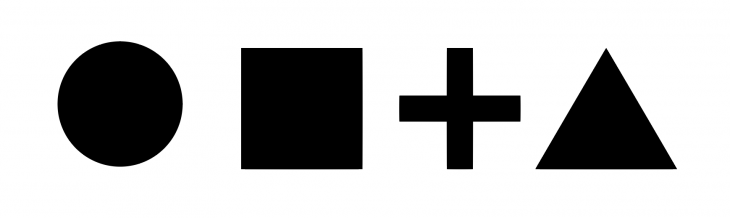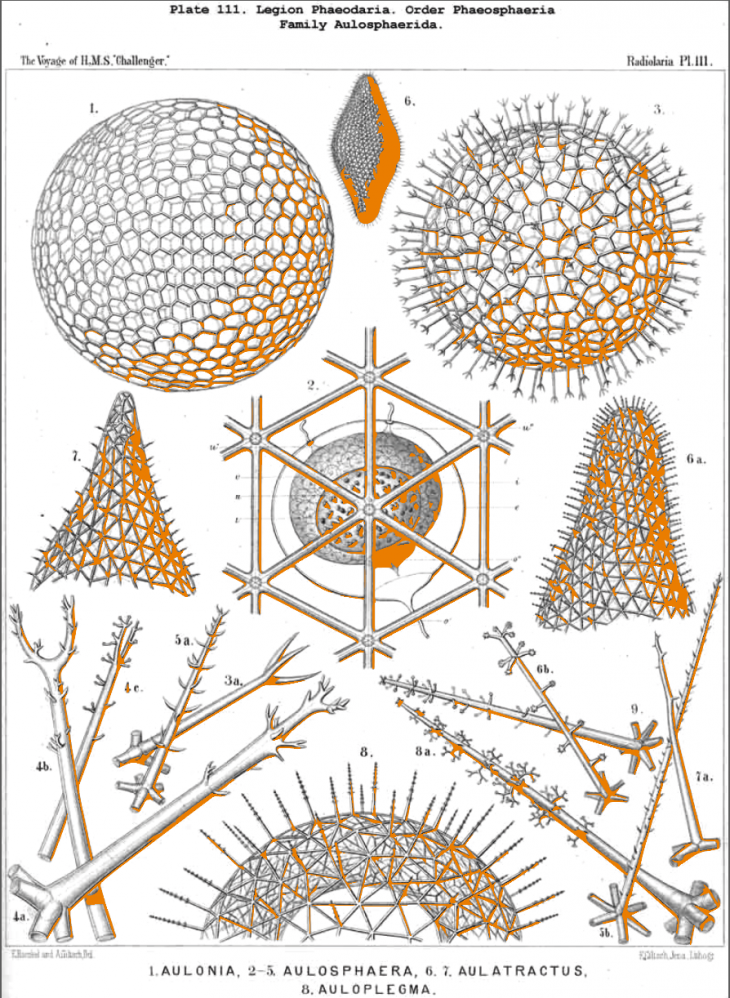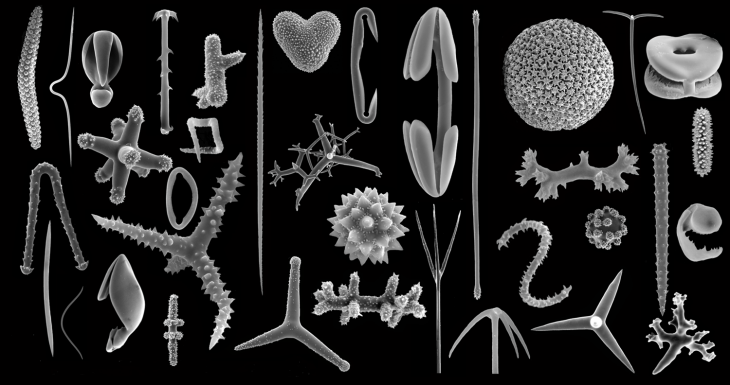MATH + BIOLOGY                                                                                                                   DESCRIBING HIMAN BEING

• This book tells us much more about potentiality of mathematical knowledge as a way of human perception.
We understand everything in logic way according to what we see, according to some simple forms, which are understandable as axiom.
• Beginning from the simplest forms we try to understand the world. Comparing the distance, we almost start to understand the relative being. “Everything is a neighbour” as was said in one book, everything is infinity. We can compare objects and

*An algebraic curve has its fundamental formula, which defines he family to which it belongs; and it’s parameters, whose quantitative variation admits of infinite variety with the limits which the formula perscribles.• At first we try to explain what dot is, than that line is a plenty of dots, than what is distance, than sinusoid as a mathematical formula of SOUND, the formula of TEM- PORARY as an integral function, and than we try to understand the formula of uni- verse.
• The main topic of this book is almost about understanding world through the prism of mathematical “axiom” of perception.

*A “principles of discontinuity”, then is inherent in all our classifications, whether mathematical, physical or biological; and the infinitude of possible forms, always limited, may be further reduced and discontinuity further revealed by imposing conditions – ..”• Searching for the essence of archetypes and the knowledge of it’s behave using 2d and 3d mathematical structures grid. This gives us the basic of the basic analogical method of architectural shapes increasing. The main goal is to understand the rules which are given us by nature.
• The compression and expansion of  grid doesn’t make the same shape, but it gives us opportunity to discover the essence of types and peaces growing, to recognise main rules of evolution developing and to understand the reason of nature multi structure being.• The most powerful tool is since always was using multidisciplinary using it in “advanced way” we have to learn it deeply, to be related with all factors in each theme, or make the collaboration between different type of knowledge, as in physics mathematic describe the function which describe physical actions.

So for understanding everything we have that kind of tools which could be precisely related with: TIME, SOUND, LIGHT, ELECTRICITY, etc. (and other physical themes of topics).